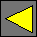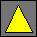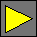April 2002 Draft JavaScript 2.0 Core Language OperatorsTuesday, October 9, 2001

## Overview

JavaScript 2.0 provides a number of operators such as such as `+`, `*`, a`[`b`]`, and `-=`. These operators are dispatched based on the types of their arguments. Some operators are dispatched only on one operand, in which case the dispatch behaves like property lookup. Other operators are dispatched on two operands simultaneously. Finally, some operators are syntactic sugars for combinations of existing operators.

There are predefined definitions for all JavaScript operators. In addition, a class may provide additional definitions by overriding the existing behavior. Such overrides apply only when at least one of the dispatched operands is an instance of that class or its descendants. Operator overriding is useful, for example, to implement units without having to add units to the core of the JavaScript 2.0 language.

This section presents the expansions of operators in JavaScript source code, the built-in definitions of those operators, and the means of overriding them.

## Invoking Operators

When an operator is invoked in an expression, the corresponding operator is invoked according to the table below. The operator invocations use pseudo-syntax to denote which operator is called; only the expression syntax on the left side of the table can be used to invoke operators.

Expression Operator Invocation
`+`x operator `"+"(`x`)`
`-`x operator `"-"(`x`)`
`~`x operator `"~"(`x`)`
`++`x `(`x` = `operator `"++"(`x`))`
x`++` `(`` = `x`, `x` = `operator `"++"(`x`), ``)`, where is a temporary variable
`--`x `(`x` = `operator `"--"(`x`))`
x`--` `(`` = `x`, `x` = `operator `"--"(`x`), ``)`, where is a temporary variable
x`(`a1`,` ...`, `an`)` operator `"()"(`x`, `a1`,` ...`, `an`)`
`new `x operator `"new"(`x`)`
`new `x`(`a1`,` ...`, `an`)` operator `"new"(`x`, `a1`,` ...`, `an`)`
x`[`a1`,` ...`, `an`]` operator `"[]"(`x`, `a1`,` ...`, `an`)`
x`[`a1`,` ...`, `an`] = `y operator `"[]="(`x`, `y`, `a1`,` ...`, `an`)`
`delete `x`[`a1`,` ...`, `an`]  ` operator `"delete[]"(`x`, `a1`,` ...`, `an`)`
x` + `y operator `"+"(`x`, `y`)`
x` - `y operator `"-"(`x`, `y`)`
x` * `y operator `"*"(`x`, `y`)`
x` / `y operator `"/"(`x`, `y`)`
x` % `y operator `"%"(`x`, `y`)`
x` << `y operator `"<<"(`x`, `y`)`
x` >> `y operator `">>"(`x`, `y`)`
x` >>> `y operator `">>>"(`x`, `y`)`
x` < `y operator `"<"(`x`, `y`)`
x` > `y operator `"<"(`y`, `x`)`
x` <= `y operator `"<="(`x`, `y`)`
x` >= `y operator `"<="(`y`, `x`)`
x` in `y operator `"in"(`x`, `y`)`
x` == `y operator `"=="(`x`, `y`)`
x` != `y `!(`operator `"=="(`x`, `y`))`
x` === `y operator `"==="(`x`, `y`)`
x` !== `y `!(`operator `"==="(`x`, `y`))`
x` & `y operator `"&"(`x`, `y`)`
x` ^ `y operator `"^"(`x`, `y`)`
x` | `y operator `"|"(`x`, `y`)`
x` += `y `(`x` = `operator `"+"(`x`, `y`))`
x` -= `y `(`x` = `operator `"-"(`x`, `y`))`
x` *= `y `(`x` = `operator `"*"(`x`, `y`))`
x` /= `y `(`x` = `operator `"/"(`x`, `y`))`
x` %= `y `(`x` = `operator `"%"(`x`, `y`))`
x` <<= `y `(`x` = `operator `"<<"(`x`, `y`))`
x` >>= `y `(`x` = `operator `">>"(`x`, `y`))`
x` >>>= `y `(`x` = `operator `">>>"(`x`, `y`))`
x` &= `y `(`x` = `operator `"&"(`x`, `y`))`
x` ^= `y `(`x` = `operator `"^"(`x`, `y`))`
x` |= `y `(`x` = `operator `"|"(`x`, `y`))`

### Operator Dispatch

Unary operators are single-dispatched just like regular methods. `in` is like a unary operator in that it can only be overridden by its right operand’s class, and it is single-dispatched by its right operand. Like in regular method dispatch, `null` is considered to be a member of only the types `Null` and `Object`.

Binary operator methods are double-dispatched based on the types of both operands. When one of the binary operators is invoked in an expression a  b, all applicable operator `"``"(`x`, `y`)` methods are examined to find the most specific one, which is then called. A definition of operator `"``"(`x`:`X`, `y`:`Y`)` is applicable to a  b if the value of a is a member of X and the value of b is a member of Y, except that, like in regular method dispatch, `null` is considered to be a member of only the types `Null` and `Object`. A definition of operator `"``"(`x`:`X`, `y`:`Y`)` is most specific if it is applicable and if every applicable definition of operator `"``"(`x`:`X'`, `y`:`Y'`)` satisfies X X' and Y Y'. If there is no most specific applicable definition of or if there is more than one most specific applicable definition of , then an error occurs when the expression a  b is evaluated.

One of the operands to an operator expression inside a class C can be a `super` expression, in which case the set of applicable definitions of the operator is restricted to those defined to take a parameter of type T in that position, where T is any proper ancestor of C. This way a method overriding an operator can invoke its superclass’s definition of that operator.

## Built-In Operator Definitions

All operators have built-in definitions. These are summarized in the table below.

Name Signature Behavior
`"()"` `(`x`:Object, ... `args`)` Call x with the arguments args and return the result.
`"new"` `(`x`:Object, ... `args`)` Call x’s constructor slot with the arguments args and return the result.
`"[]"` `(`x`:Object, `n`:Object)` Return the value of x’s most derived, `public`, indexable property named n`.toString()`. See property lookup.
`"[]="` `(`x`:Object, `y`:Object, `n`:Object)` Set the value of x’s most derived, `public`, indexable property named n`.toString()` to y.
`(`x`:Array, `y`:Object, `n`:Object)` Set the value of x’s most derived, `public`, indexable property named n`.toString()` to y. Update the `length` property as in JavaScript 1.5.
`"delete[]"` `(`x`:Object, `n`:Object)` Try to delete x’s most derived, `public`, indexable property named n`.toString()`.
`"~"` `(`x`:Object)` Return the bitwise complement of ToInt32(operator `"+"(`x`)`).
`"++"` `(`x`:Object)` Return operator `"+"(`operator `"+"(`x`), 1)`.
`"--"` `(`x`:Object)` Return operator `"-"(`operator `"+"(`x`), 1)`.
`"+"` `(`x`:Object)` Return ToNumber(x).
`(`x`:Object, `y`:Object)` Let x' = ToPrimitive(x) and y' = ToPrimitive(y). If at least one of x' and y' is a string, then return operator `"+"(`x'`, `y'`)`. Otherwise return ToNumber(x') + ToNumber(y').
`(`x`:String, `y`:Object)` Return x concatenated with y`.toString()`.
`(`x`:Object, `y`:String)` Return x`.toString()` concatenated with y.
`(`x`:String, `y`:String)` Return x concatenated with y.
`(`x`:Number, `y`:Number)` Return x + y.
`"-"` `(`x`:Object)` Return -ToNumber(x).
`(`x`:Object, `y`:Object)` Return ToNumber(x) – ToNumber(y).
`"*"` `(`x`:Object, `y`:Object)` Return ToNumber(x ToNumber(y).
`"/"` `(`x`:Object, `y`:Object)` Return ToNumber(x) / ToNumber(y).
`"%"` `(`x`:Object, `y`:Object)` Return ToNumber(x) % ToNumber(y).
`"<<"` `(`x`:Object, `y`:Object)` Return ToNumber(x) << ToNumber(y).
`">>"` `(`x`:Object, `y`:Object)` Return ToNumber(x) >> ToNumber(y).
`">>>"` `(`x`:Object, `y`:Object)` Return ToNumber(x) >>> ToNumber(y).
`"<"` `(`x`:Object, `y`:Object)` Same as in JavaScript 1.5.
`">="` `(`x`:Object, `y`:Object)` Same as in JavaScript 1.5.
`"in"` `(`x`:Object, `y`:Object)` Same as in JavaScript 1.5.
`"=="` `(`x`:Object, `y`:Object)` Same as in JavaScript 1.5.
`"==="` `(`x`:Object, `y`:Object)` Same as in JavaScript 1.5.
`"&"` `(`x`:Object, `y`:Object)` Return ToNumber(x) & ToNumber(y).
`"^"` `(`x`:Object, `y`:Object)` Return ToNumber(x) ^ ToNumber(y).
`"|"` `(`x`:Object, `y`:Object)` Return ToNumber(x) | ToNumber(y).

## Operator Overriding

An operator can be overridden only inside a class C. That class should define a function with the `operator` attribute. The function’s name must be one of the strings in the table below. The table also defines the acceptable signatures that the operator can have. At least one parameter must be specified as taking a value of type C (a type that is a subclass of C is not acceptable). If the operator is `===`, then both parameters must take values of type C. A class can define several different signatures of the same binary (but not unary) operator, differing in the choice of parameter types X or Y, which may be omitted if they are `Object`. The parameters denoted as taking types C, X, or Y in the table below cannot be optional or named parameters. The return type of an operator override can be any type.

Name Signatures
```"~" "++" "--"``` `(`x`:`C`)`
```"+" "-"``` `(`x`:`C`)`
`(`x`:`C`, `y`:`Y`)`
`(`x`:`X`, `y`:`C`)`
`(`x`:`C`, `y`:`C`)`
```"*" "/" "%" "<<" ">>" ">>>" "<" "<=" "==" "&" "^" "|"``` `(`x`:`C`, `y`:`Y`)`
`(`x`:`X`, `y`:`C`)`
`(`x`:`C`, `y`:`C`)`
`"==="` `(`x`:`C`, `y`:`C`)`
`"in"` `(`x`:`X`, `y`:`C`)`
```"()" "new" "[]" "delete[]"``` `(`x`:`C`, `a1`,` ...`, `an`)`
`"[]="` `(`x`:`C`, `y`:`Y`, `a1`,` ...`, `an`)`

The operator methods should return the result of the operator. For the `++` and `--` operators, the result is the incremented or decremented value. The `()`, `new`, `[]`, `delete[]`, and `[]=` operators take additional argument lists. If desired, these argument lists can include optional, named, or rest arguments. The `[]=` operator must take one more initial required parameter, which is the value stored in the element.

The `>`, `>=`, `!=`, and `!==` operators cannot be overridden directly because they are syntactic sugars for invocations of the `<`, `<=`, `==`, and `===` operators; override the latter operators instead. The `!`, `||`, `^^`, `&&`, and `?:` operators cannot be overridden directly, but they are affected by any redefinition of `toBoolean`. See rationale.

Definitions of operators cannot specify a namespace. No access restrictions can be imposed on operators. Operators cannot be defined in class extensions.

### Example

The following class illustrates overriding a few operators.

```class Complex {
var x:Number, y:Number;

operator function "+"(a:Complex):Complex {
return a;
}

operator function "-"(a:Complex):Complex {
return new Complex(x: -a.x, y: -a.y);
}

operator function "+"(a:Complex, b:Complex):Complex {
return new Complex(x: a.x+b.x, y: a.y+b.y);
}

operator function "+"(a:Complex, b:Number):Complex {
return new Complex(x: a.x+b, y: a.y);
}

operator function "+"(a:Number, b:Complex):Complex {
return new Complex(x: a+b.x, y: b.y);
}

function toBoolean():Boolean {
return this.x != 0 || this.y != 0;
}
}```

## `toBoolean` Method

Just like `toString`, every object has a `toBoolean` method that can be overridden. This method is called by statements such as `if`, `while`, `do while`, and `for` and operators such as `!`, `||`, `^^`, `&&`, and `? :`. This method should return either `true` or `false`.

## For-In Operator

An object x’s class can override the meaning of for-in loops such as `for (`v` in `x`)` by overriding the following three methods which are located in the system-defined namespace `Iterator`:

Method Description
x`.Iterator::forIn()` If the iteration is empty, return `null`. Otherwise, return an object o with `public` properties named `value` and `state` (o may also have other properties). The value of o`.value` is the first element returned by the iteration. The value of o`.state` can have any type and will be passed exactly once to either `Iterator::next` or `Iterator::done`. The value of o is not guaranteed to be valid after o`.state` is passed to `Iterator::next` or `Iterator::done`.
x`.Iterator::next(`i`)` i was returned by a previous call to `Iterator::forIn` or `Iterator::next`. If the iteration is done, return `null`. Otherwise, return an object o with `public` properties named `value` and `state` (o may also have other properties). The value of o`.value` is the next element returned by the iteration. The value of o`.state` can have any type and will be passed exactly once to either `Iterator::next` or `Iterator::done`. The value of o is not guaranteed to be valid after o`.state` is passed to `Iterator::next` or `Iterator::done`.
x`.Iterator::done(`i`)` i was returned by a previous call to `Iterator::forIn` or `Iterator::next`. This call alerts x that the iteration is exiting prematurely and it can do whatever cleanup it needs on i. Every i returned by `Iterator::forIn` or `Iterator::next` is guaranteed to be subsequently passed exactly once either to `Iterator::next` or `Iterator::done`. `Iterator::done` should return `undefined`.

 Waldemar Horwat Last modified Tuesday, October 9, 2001